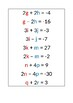# Substitution Simultaneous EquationsSubject
Resource Type
Product Rating
File Type

Word Document File

(12 KB|3 pages)
Product Description

This activity is designed for students who have learnt the algebraic substitution method of solution for linear simultaneous equations but not the elimination method.

Students will be handed one equation with two variables and be asked to find the person in the class who has another equation with the same variables and these pairs will then solve their pair of simultaneous equations together using the algebraic substitution method. All of the pairs of equations involve rearranging one of the equations and then multiplying this when substituting it so students will find the task moderately challenging and this will generate interest for an easier solution. The teacher will then introduce the elimination method as an easier solution method for when the variables have matching coefficients.

Alternatively, this activity could be used as a diagnostic test for the students using any solution method for simultaneous equations.

Total Pages
3 pages
Included
Teaching Duration
N/A
Report this Resource to TpT
Reported resources will be reviewed by our team. Report this resource to let us know if this resource violates TpT’s content guidelines.